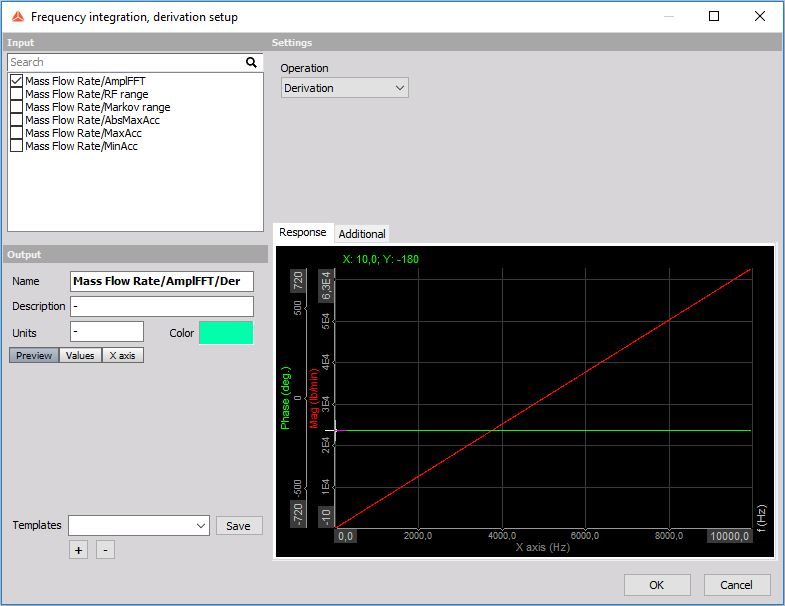# Frequency integration and derivation

When opening a new Frequency integration, derivation math function the following setup window will appear:Under settings we can select the type of operation integral functions and derivative funtions.

Integration and double integration

• Integration is used for functions where the carrier of information is the DC value (the offset) or the dynamic part of the signal.

• Double integration is used for dynamic signals

Derivation and double derivation * Derivation * Double derivation